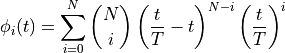# control.flatsys.BezierFamily¶

class control.flatsys.BezierFamily(N, T=1)[source]

Bases: BasisFamily

Bezier curve basis functions.

This class represents the family of polynomials of the formParameters
• N (int) – Degree of the Bezier curve.

• T (float) – Final time (used for rescaling).

Methods

 eval Compute function values given the coefficients and time points. eval_deriv Evaluate the kth derivative of the ith basis function at time t. var_ncoefs Get the number of coefficients for a variable
__call__(i, t, var=None)[source]

Evaluate the ith basis function at a point in time

eval(coeffs, tlist, var=None)[source]

Compute function values given the coefficients and time points.

eval_deriv(i, k, t, var=None)[source]

Evaluate the kth derivative of the ith basis function at time t.

var_ncoefs(var)[source]

Get the number of coefficients for a variable